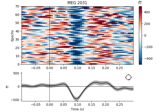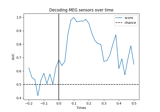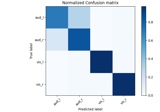# mne.preprocessing.Xdawn#

class mne.preprocessing.Xdawn(n_components=2, signal_cov=None, correct_overlap='auto', reg=None)[source]#

Implementation of the Xdawn Algorithm.

Xdawn  is a spatial filtering method designed to improve the signal to signal + noise ratio (SSNR) of the ERP responses. Xdawn was originally designed for P300 evoked potential by enhancing the target response with respect to the non-target response. This implementation is a generalization to any type of ERP.

Parameters:
n_components`int`, (default 2)

The number of components to decompose the signals.

signal_cov`None` | `Covariance` | `ndarray`, shape (n_channels, n_channels)

(default None). The signal covariance used for whitening of the data. if None, the covariance is estimated from the epochs signal.

correct_overlap‘auto’ or bool (default ‘auto’)

Compute the independent evoked responses per condition, while correcting for event overlaps if any. If ‘auto’, then overlapp_correction = True if the events do overlap.

reg

If not None (same as `'empirical'`, default), allow regularization for covariance estimation. If float, shrinkage is used (0 <= shrinkage <= 1). For str options, `reg` will be passed as `method` to `mne.compute_covariance()`.

Notes

New in version 0.10.

References

Attributes:
filters_

If fit, the Xdawn components used to decompose the data for each event type, else empty. For each event type, the filters are in the rows of the corresponding array.

patterns_

If fit, the Xdawn patterns used to restore the signals for each event type, else empty.

evokeds_

If fit, the evoked response for each event type.

event_id_`dict`

The event id.

correct_overlap_bool

Whether overlap correction was applied.

Methods

 `apply`(inst[, event_id, include, exclude]) Remove selected components from the signal. `fit`(epochs[, y]) Fit Xdawn from epochs. `fit_transform`(X[, y]) Fit to data, then transform it. `get_params`([deep]) Get parameters for this estimator. Not implemented, see Xdawn.apply() instead. `set_params`(**params) Set the parameters of this estimator. `transform`(inst) Apply Xdawn dim reduction.
apply(inst, event_id=None, include=None, exclude=None)[source]#

Remove selected components from the signal.

Given the unmixing matrix, transform data, zero out components, and inverse transform the data. This procedure will reconstruct the signals from which the dynamics described by the excluded components is subtracted.

Parameters:
instinstance of `Raw` | `Epochs` | `Evoked`

The data to be processed.

event_id

The kind of event to apply. if None, a dict of inst will be return one for each type of event xdawn has been fitted.

include

The indices referring to columns in the ummixing matrix. The components to be kept. If None, the first n_components (as defined in the Xdawn constructor) will be kept.

exclude

The indices referring to columns in the ummixing matrix. The components to be zeroed out. If None, all the components except the first n_components will be exclude.

Returns:
out`dict`

A dict of instance (from the same type as inst input) for each event type in event_id.

Examples using `apply`:XDAWN Denoising

XDAWN Denoising
fit(epochs, y=None)[source]#

Fit Xdawn from epochs.

Parameters:
epochsinstance of `Epochs`

An instance of Epoch on which Xdawn filters will be fitted.

y

If None, used epochs.events[:, 2].

Returns:
selfinstance of `Xdawn`

The Xdawn instance.

Examples using `fit`:XDAWN Denoising

XDAWN Denoising
fit_transform(X, y=None, **fit_params)[source]#

Fit to data, then transform it.

Fits transformer to `X` and `y` with optional parameters `fit_params`, and returns a transformed version of `X`.

Parameters:
X`array`, shape (n_samples, n_features)

Training set.

y`array`, shape (n_samples,)

Target values or class labels.

**fit_params`dict`

Additional fitting parameters passed to the `fit` method..

Returns:
X_new`array`, shape (n_samples, n_features_new)

Transformed array.

get_params(deep=True)[source]#

Get parameters for this estimator.

Parameters:
deepbool, optional

If True, will return the parameters for this estimator and contained subobjects that are estimators.

Returns:
params`dict`

Parameter names mapped to their values.

inverse_transform()[source]#

set_params(**params)[source]#

Set the parameters of this estimator.

The method works on simple estimators as well as on nested objects (such as pipelines). The latter have parameters of the form `<component>__<parameter>` so that it’s possible to update each component of a nested object.

Parameters:
**params`dict`

Parameters.

Returns:
instinstance

The object.

transform(inst)[source]#

Apply Xdawn dim reduction.

Parameters:
instEpochs | Evoked | ndarray, shape ([n_epochs, ]n_channels, n_times)

Data on which Xdawn filters will be applied.

Returns:
Xndarray, shape ([n_epochs, ]n_components * n_event_types, n_times)

Spatially filtered signals.

## Examples using `mne.preprocessing.Xdawn`#Decoding (MVPA)

Decoding (MVPA)XDAWN Denoising

XDAWN DenoisingXDAWN Decoding From EEG data

XDAWN Decoding From EEG data